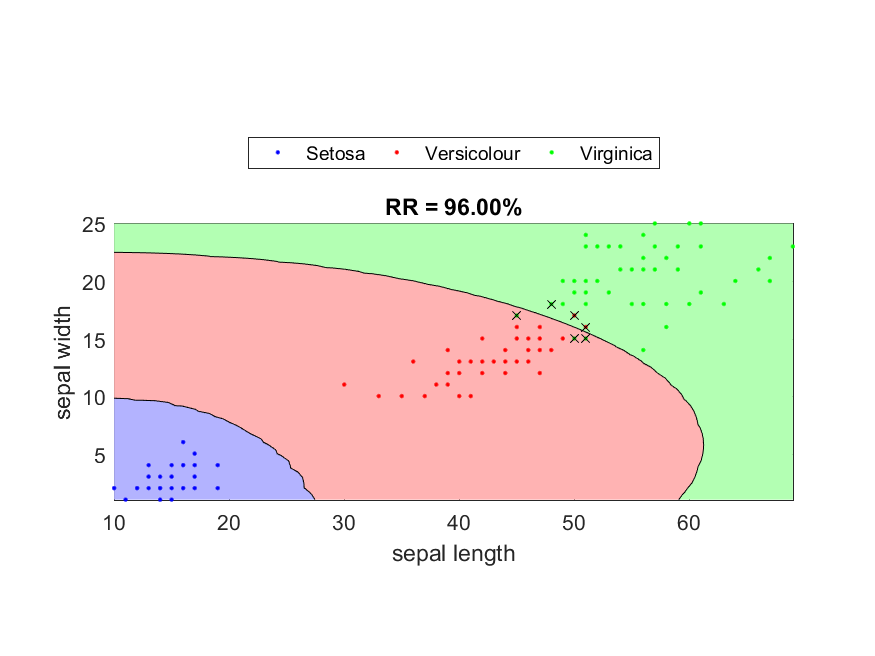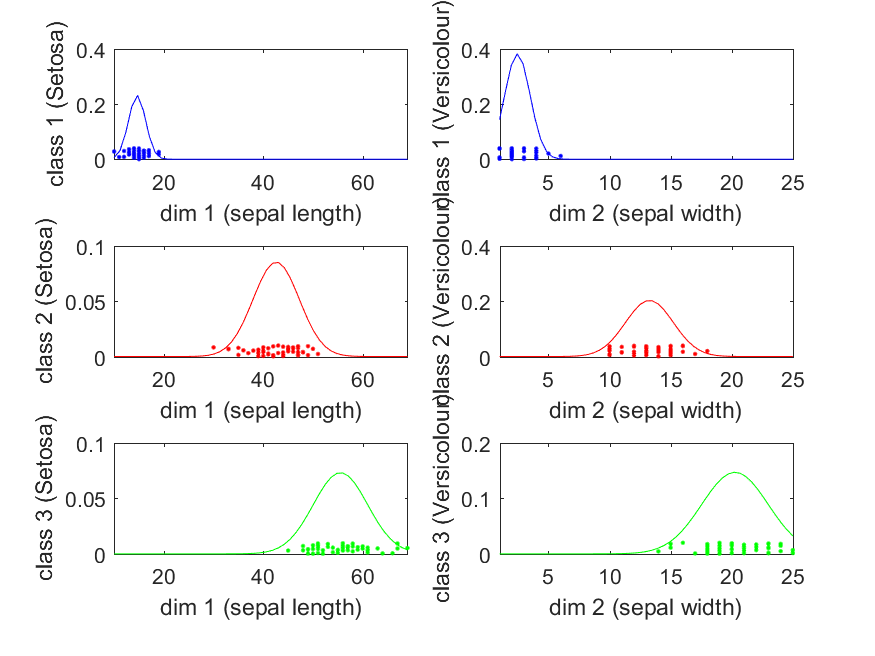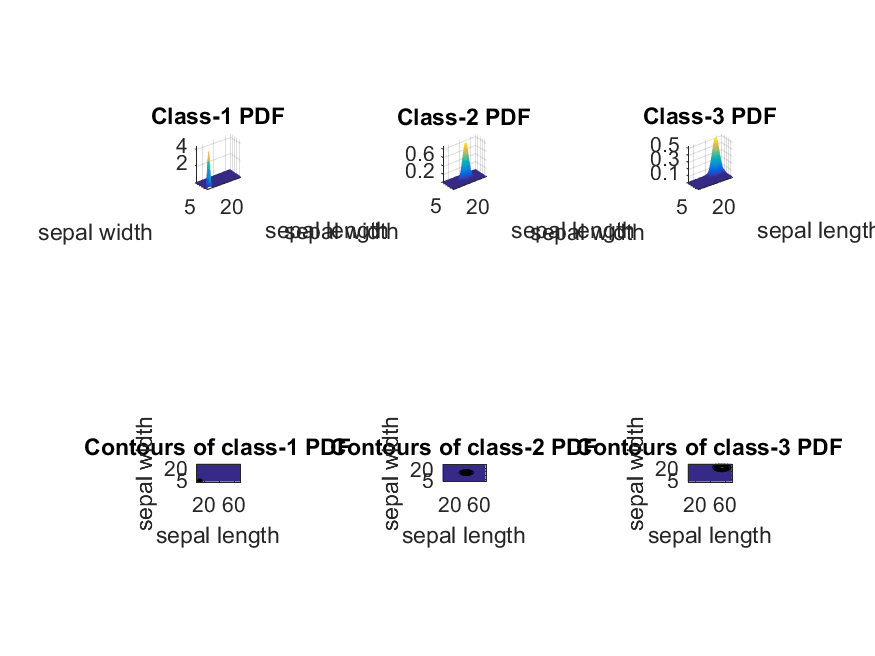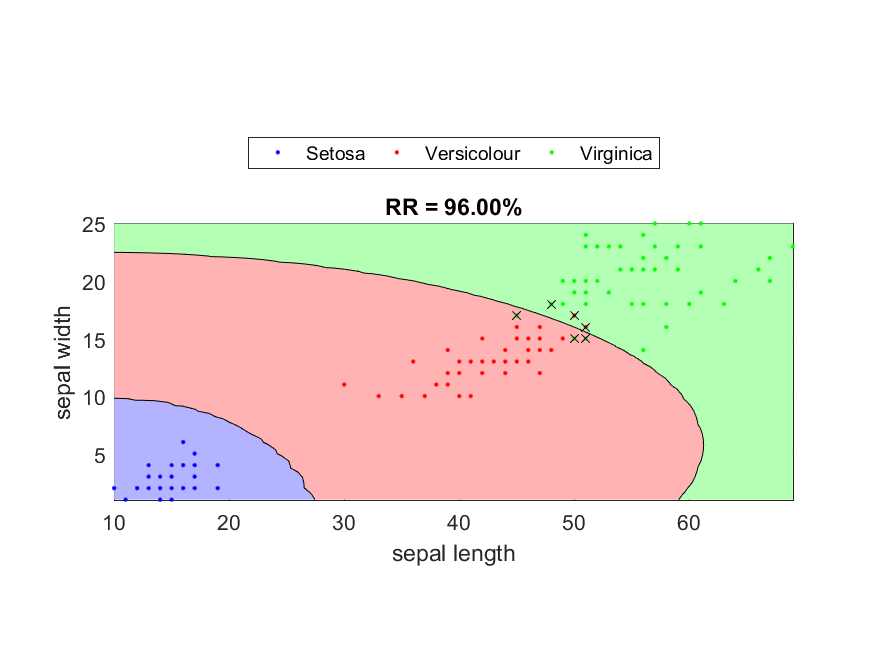5-5 Naive Bayes Classifiers (嚙踝蕭嚙踝蕭嚙踝蕭嚙踝蕭嚙踝蕭嚙踝蕭嚙踝蕭嚙踝蕭嚙踝蕭嚙踝蕭嚙?

[chinese][all]

If we assume that the features of the given dataset are independent, then we can perform PDF modeling on each dimension sequentially. The final PDF for each class is then given by the multiplication of the PDF of the same class over each dimension. In symbols, we assume that where X = [X1, X2, ..., Xd] is a feature vector and C is a class. Despite the assumption seems too strong for real-world data, the resulting naive Bayes classifier (NBC for short) is highly successful in many practical applications.

p(X|C) = P(X1|C)P(X2|C) ... P(Xd|C)

where X = [X1, X2, ..., Xd] is a feature vector and C is a class. Despite the assumption seems too strong for real-world data, the resulting naive Bayes classifier (NBC for short) is highly successful in many practical applications.

In practice, we can apply 1D Gaussian functions for modeling the data of a specific class and a specific dimension. The training of a NBC can be summarized as follows:

1. Assume that data in class i and dimension j is governed by a 1-dimensional Gaussian PDF:
g(x, mi, si2) = [(2p)*si2]-0.5*exp[-(x-mi)2)/(2si2)]
where mi is the mean value and si2 is the variance of the PDF. Given the dimension-j training data {x1, x2, ... xn} in class i, the optimum parameters in the sense of maximum likelihood can be expressed as follows:
mi = (x1+x2+...+xn)/n
si2 = [ (x1-mi)2 + (x2-mi)2 + ... + (xn-mi)2 ] /(n-1)
2. Since the Gaussian PDF does not reflect the prior probability of class i (denoted by p(ci)), we usually use p(ci)g(x, mi, Si) to represent the probability density of a given vector x. In practice, p(ci) is computed as the number of entries of class i divided by the number of total entries in the training set.
3. In application, for a given vector x of unknown class, the higher the probability density p(ci)g(x, mi, Si) is, the more likelihood that this vector belongs to class i.

When implementing the classifier, usually we do not calculate p(ci)g(x, mi, Si) directly since the exponential function is likely to introduce round-off errors. Instead, we compute the natural logarithm of the probability density, as follows:

log[p(ci)g(x, mi, Si)] = log(p(ci)) - (d*log(2p) + log|Si|)/2 - (x-mi)TSi-1(x-mi)/2
The decision boundary between class i and j is represented by the following trajectory:
p(ci)g(x, mi, Si) = p(cj)g(x, mj, Sj).
Taking the logrithm of both sides, we have
log(p(ci)) - (d*log(2p) + log|Si|)/2 - (x-mi)TSi-1(x-mi)/2 = log(p(cj)) - (d*log(2p) + log|S|j)/2 - (x-mj)TSj-1(x-mj)/2
After simplification, we have the decision boundary as the following equation:
(x-mj)TSj-1(x-mj) - (x-mi)TSi-1(x-mi) = log{[|S|i p2(ci)]/[|S|j p2(cj)]}
where the right-hand side is a constant. Since both (x-mj)TSj-1(x-mj) and (x-mi)TSi-1(x-mi) are quadratic, the above equation represents a decision boundary of the quadratic form in the d-dimensional feature space.

In the following example, we shall use dimensions 3 and 4 of the Iris dataset for NBC:

Example 1: nbc01dataPlot.mDS = prData('iris'); DS.input=DS.input(3:4, :); % Only take dimensions 3 and 4 for 2d visualization plotOpt=1; % Plotting [nbcPrm, logLike, recogRate, hitIndex]=nbcTrain(DS, [], plotOpt); fprintf('Recog. rate = %f%%\n', recogRate*100); Recog. rate = 96.000000%In the above example, each misclassified data point is labeled with a cross. Moreover, we use the number of entries of each class as the class prior probability for computing the probability density. In general, there are two ways to specify class prior probabilities:

• Use the number of entries in each class as the prior probabilities. You can divide the total number of entries in the training set to have a real probability between 0 and 1. In practice, the division does not change the classification results since it is applied to every class' PDF. (You can use the function dsClassSize.m to find the number of entries in each class.)
• If we have the domain knowledge of the prior probabilities, we can assign them directly.

We can plot the 1d PDFs for all classes and dimensions, together with the corresponding data points, as follows:

Example 2: nbcPlot00.mDS=prData('iris'); DS.input=DS.input(3:4, :); [nbcPrm, logLike, recogRate, hitIndex]=nbcTrain(DS); nbcPlot(DS, nbcPrm, '1dPdf');For the above example, we can go one step further to plot the class-specific PDFs as 3D surfaces and display the corresponding contours, as follows:

Example 3: nbcPlot01.mDS=prData('iris'); DS.input=DS.input(3:4, :); [nbcPrm, logLike, recogRate, hitIndex]=nbcTrain(DS); nbcPlot(DS, nbcPrm, '2dPdf');Based on the computed PDF for each class, we can plot the decision boundaries, as follows:

Example 4: nbcPlot02.mDS=prData('iris'); DS.input=DS.input(3:4, :); [nbcPrm, logLike, recogRate, hitIndex]=nbcTrain(DS); DS.hitIndex=hitIndex; % Attach hitIndex to DS for plotting nbcPlot(DS, nbcPrm, 'decBoundary');Data Clustering and Pattern Recognition (資料分群與樣式辨認)